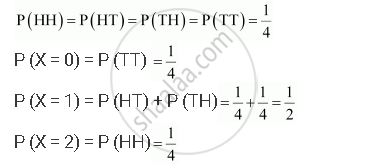Share

# Find the Probability Distribution Of Number of Heads in Two Tosses of a Coin - CBSE (Science) Class 12 - Mathematics

ConceptRandom Variables and Its Probability Distributions

#### Question

Find the probability distribution of number of heads in two tosses of a coin

#### Solution

When one coin is tossed twice, the sample space is

{HH, HT, TH, TT}

Let X represent the number of heads.

∴ X (HH) = 2, X (HT) = 1, X (TH) = 1, X (TT) = 0

Therefore, X can take the value of 0, 1, or 2.

It is known that,Thus, the required probability distribution is as follows.

 X 0 1 2 P(X) 1/4 1/2 1/4
Is there an error in this question or solution?

#### Video TutorialsVIEW ALL 

Solution Find the Probability Distribution Of Number of Heads in Two Tosses of a Coin Concept: Random Variables and Its Probability Distributions.
S